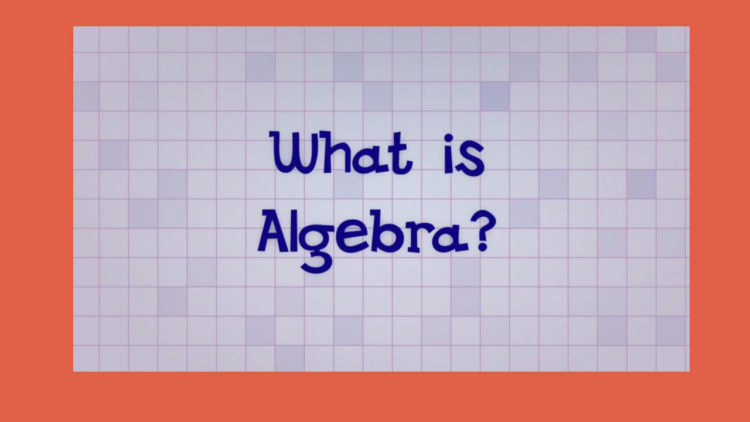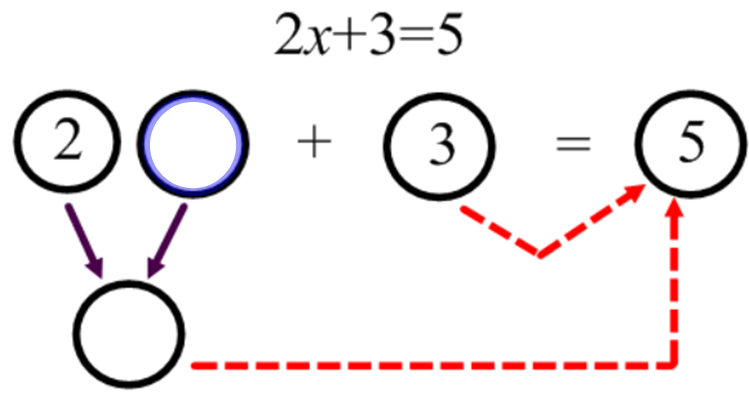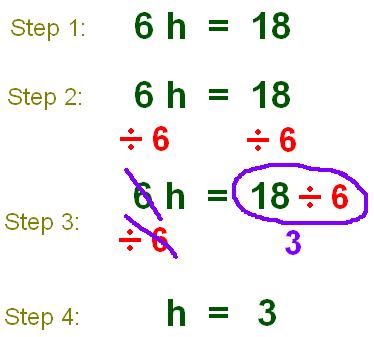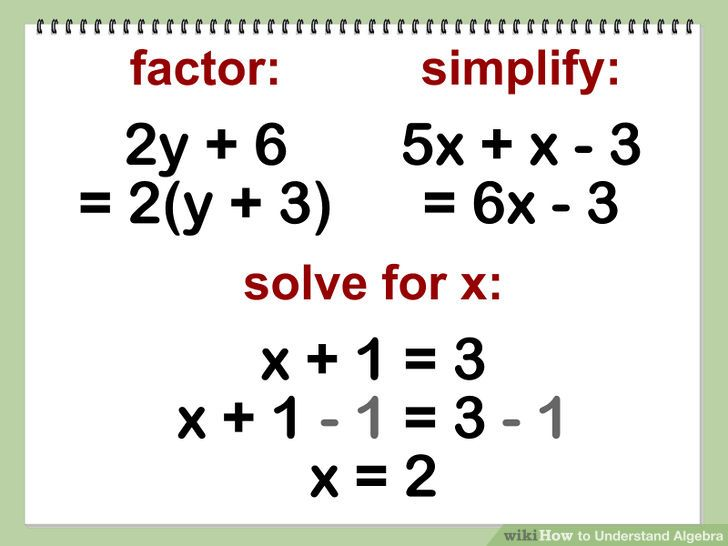# What is Algebra? (Exposed)!Well, What is Algebra??? In my opinion is that, Algebra is nothing but solving for a unknown number.. I can show u some example's !!!!! But all Algebra is the part of mathematics in which letters and other general symbols are used to represent numbers and quantities in formula and equations.

5x=25 (This would represent as Algebra. U know why? The "x" would be the unknown number in this equation or such a expression)!!!In this equation, the unknown number would be "2x" or just "x" Well with this equation,there is solution which would be x=1 how???

Well U have 2x+3=5. All mathematics's would know that we need to isolate 2x so we would subtract 3 to have 2x by it self on 1 side and the difference on the other

2x=2 so as other's we would divide to get just "x" by it self 2x/2=2/2 to have

x=1

For any reason u dont understand this equation I did 2x+3=5 here's 2 more problem but with the steps shown!!!But with this problem it's showed the simplify version but both answer will be the same!!! But place in a y-term versionNote by Noah Smalls
4 years, 3 months ago

This discussion board is a place to discuss our Daily Challenges and the math and science related to those challenges. Explanations are more than just a solution — they should explain the steps and thinking strategies that you used to obtain the solution. Comments should further the discussion of math and science.

When posting on Brilliant:

• Use the emojis to react to an explanation, whether you're congratulating a job well done , or just really confused .
• Ask specific questions about the challenge or the steps in somebody's explanation. Well-posed questions can add a lot to the discussion, but posting "I don't understand!" doesn't help anyone.
• Try to contribute something new to the discussion, whether it is an extension, generalization or other idea related to the challenge.
• Stay on topic — we're all here to learn more about math and science, not to hear about your favorite get-rich-quick scheme or current world events.

MarkdownAppears as
*italics* or _italics_ italics
**bold** or __bold__ bold
- bulleted- list
• bulleted
• list
1. numbered2. list
1. numbered
2. list
Note: you must add a full line of space before and after lists for them to show up correctly
paragraph 1paragraph 2

paragraph 1

paragraph 2

[example link](https://brilliant.org)example link
> This is a quote
This is a quote
    # I indented these lines
# 4 spaces, and now they show
# up as a code block.

print "hello world"
# I indented these lines
# 4 spaces, and now they show
# up as a code block.

print "hello world"
MathAppears as
Remember to wrap math in $$ ... $$ or $ ... $ to ensure proper formatting.
2 \times 3 $2 \times 3$
2^{34} $2^{34}$
a_{i-1} $a_{i-1}$
\frac{2}{3} $\frac{2}{3}$
\sqrt{2} $\sqrt{2}$
\sum_{i=1}^3 $\sum_{i=1}^3$
\sin \theta $\sin \theta$
\boxed{123} $\boxed{123}$

## Comments

Sort by:

Top Newest

Cool

- 4 years, 3 months ago

Log in to reply

×

Problem Loading...

Note Loading...

Set Loading...